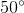ISEE Lower Level Quantitative : Shape Properties

Example Questions

← Previous 1 3

Example Question #1 : Shape Properties

Which of the following shapes is NOT a quadrilateral?

Triangle

Square

Rectangle

Kite

Rhombus

Triangle

Explanation:

A quadrilateral is any two-dimensional shape withsides. The only shape listed that does not havesides is a triangle.

Example Question #2 : How To Find The Area Of A Parallelogram

What is the main difference between a square and a rectangle?

The number of sides they each have

Their angle measurments

Their side lengths

Their color

The sum of their angles

Their side lengths

Explanation:

The only difference between a rectangle and a square is their side lengths. A square has to haveequal side lengths, but the opposite side lengths of a rectangle only have to be equal.

Example Question #1 : Shape Properties

What two shapes can a square be classified as?

Trapezoid and Triangle

Rhombus and Triangle

Trapezoid and Rhombus

Rectangle and Rhombus

Rectangle and Triangle

Rectangle and Rhombus

Explanation:

A square can also be a rectangle and a rhombus because a rectangle has to have at leastsets of equal side lengths and a rhombus has to haveequal side lengths, like a square, and at leastsets of equal angles.

Example Question #3 : How To Find The Area Of A Parallelogram

What is the main difference between a triangle and a rectangle?

The volume

The length of the sides

The area

The color

The number of sides

The number of sides

Explanation:

Out of the choices given, the only characteristic used to describe shapes is the number of sides. A triangle hassides and a rectangle hassides.

Example Question #4 : How To Find The Area Of A Parallelogram

Which two shapes have to haveright angles?

Square and Rectangle

Rectangle and Rhombus

Square and Rhombus

Square and Parallelogram

Rectangle and Parallelogram

Square and Rectangle

Explanation:

By definition, the only two quadrilaterals that have to haveright angles, are the square and the rectangle.

Example Question #1 : Understand Categories And Subcategories Of Two Dimensional Figures: Ccss.Math.Content.5.G.B.3

Which of the shapes is NOT a quadrilateral?

Rhombus

Rectangle

Hexagon

Square

Trapezoid

Hexagon

Explanation:

A quadrilateral is asided shape. The only shape listed that does not havesides is a hexagon, which hassides.

Example Question #3 : Understand Categories And Subcategories Of Two Dimensional Figures: Ccss.Math.Content.5.G.B.3

Which of the following shapes is NOT a parallelogram?

Rhombus

Square

Rectangle

Kite

Kite

Explanation:

A rectangle, square, and rhombus can all be classified as a parallelogram because each shape has opposite side lengths that are equal. A kite does not.

Example Question #2 : Shape Properties

What is the difference between a trapezoid and a isosceles trapezoid?

An isosceles trapezoid has to have equal base angles

An isosceles trapezoid has to have equal side lengths

A trapezoid has to have equal base angles

A trapezoid has to have equal side lengths

There is no difference between them

An isosceles trapezoid has to have equal base angles

Explanation:

By definition, an isosceles trapezoid has to haveequal base angles, but a trapezoid does not have to have equal angles.

Example Question #1 : Shape Properties

Which shape is NOT a quadrilateral?

Circle

Trapezoid

Kite

Rhombus

Rectangle

Circle

Explanation:

A quadrilateral has to havesides, a circle does not have any sides.

Example Question #2 : How To Find If Right Triangles Are Similar

What is the main difference between a right triangle and an isosceles triangle?

An isosceles triangle has to have aangle and a right triangle has to haveequal, base angles.

A right triangle has to have aangle and an isosceles triangle has to haveequal, base angles.

A right triangle has to have aangle and an isosceles triangle has to haveequal, base angles.

A right triangle has to have aangle and an isosceles triangle has to haveequal, base angles.

A right triangle has to have aangle and an isosceles triangle has to haveequal, base angles.

A right triangle has to have aangle and an isosceles triangle has to haveequal, base angles.
By definition, a right triangle has to have one right angle, or aangle, and an isosceles triangle hasequal base angles and two equal side lengths.